## Predictive Analysis, Binary Classification (Cookbook)

This notebook contains my notes for Predictive Analysis on Binary Classification. It acts as a cookbook.

# Importing and sizing up a New Data Set

The file is comma delimited, with the data for one experiment occupying one line of text. This makes it a simple matter to read a line, split it on the comma delimiters, and stack the resulting lists into an outer list containing the whole data set.
In :
```import urllib
from urllib.request import urlopen
import sys
#read data from uci data repository
target_url = urllib.request.Request("https://archive.ics.uci.edu/ml/machine-learning-databases/undocumented/connectionist-bench/sonar/sonar.all-data")
data = urlopen(target_url)

#arrange data into list for labels and list of lists for attributes
xList = []
labels = []
for line in data:
#split on comma
row = line.strip().decode().split(",")
xList.append(row)
sys.stdout.write("Number of Rows of Data = " + str(len(xList)) + '\n')
sys.stdout.write("Number of Columns of Data = " + str(len(xList)))
```
```Number of Rows of Data = 208
Number of Columns of Data = 61```

If the data set has many more columns than rows, you may be more likely to get the best prediction with penalized linear regression and vice versa.

# Determining the Nature of Attributes

The next step in the checklist is to determine how many of the columns of data are numeric versus categorical

In :
```#arrange data into list for labels and list of lists for attributes

nrow = len(xList)
ncol = len(xList)

type = *3
colCounts = []

for col in range(ncol):
for row in xList:
try:
a = float(row[col])
if isinstance(a, float):
type += 1
except ValueError:
if len(row[col]) > 0:
type += 1
else:
type += 1

colCounts.append(type)
type = *3

sys.stdout.write("Col#" + '\t' + "Number" + '\t' + "Strings" + '\t ' + "Other\n")
iCol = 0
#I deleted one /t to make it align.
for types in colCounts:
sys.stdout.write(str(iCol) + '\t' + str(types) + '\t' +
str(types) + '\t' + str(types) + "\n")
iCol += 1
```
```Col#	Number	Strings	 Other
0	208	0	0
1	208	0	0
2	208	0	0
3	208	0	0
4	208	0	0
5	208	0	0
6	208	0	0
7	208	0	0
8	208	0	0
9	208	0	0
10	208	0	0
11	208	0	0
12	208	0	0
13	208	0	0
14	208	0	0
15	208	0	0
16	208	0	0
17	208	0	0
18	208	0	0
19	208	0	0
20	208	0	0
21	208	0	0
22	208	0	0
23	208	0	0
24	208	0	0
25	208	0	0
26	208	0	0
27	208	0	0
28	208	0	0
29	208	0	0
30	208	0	0
31	208	0	0
32	208	0	0
33	208	0	0
34	208	0	0
35	208	0	0
36	208	0	0
37	208	0	0
38	208	0	0
39	208	0	0
40	208	0	0
41	208	0	0
42	208	0	0
43	208	0	0
44	208	0	0
45	208	0	0
46	208	0	0
47	208	0	0
48	208	0	0
49	208	0	0
50	208	0	0
51	208	0	0
52	208	0	0
53	208	0	0
54	208	0	0
55	208	0	0
56	208	0	0
57	208	0	0
58	208	0	0
59	208	0	0
60	0	208	0
```

The code runs down each column and adds up the number of entries that are numeric (int or float), the number of entries that are non-empty strings, and the number that are empty. The result is that the first 60 columns contain all numeric values and the last column contains all strings. The string values are the labels.

Generally, categorical variables are presented as strings, as in this example. In some cases, binary‐valued categorical variables are presented as a 0,1 numeric variable.

###### After determining which attributes are categorical and which are numeric, you’ll

want some descriptive statistics for the numeric variables and a count of the unique categories in each categorical attribute.

# Summary Statistics for Numeric and Categorical Attributes

In :
```import numpy as np
#generate summary statistics for column 3 (e.g.)
col = 3
colData = []
for row in xList:
colData.append(float(row[col]))

colArray = np.array(colData)
colMean = np.mean(colArray)
colsd = np.std(colArray)
sys.stdout.write("Mean = " + '\t' + str(colMean) + '\t\t' +
"Standard Deviation = " + '\t ' + str(colsd) + "\n")

#calculate quantile boundaries
ntiles = 4

percentBdry = []

for i in range(ntiles+1):
percentBdry.append(np.percentile(colArray, i*(100)/ntiles))

sys.stdout.write("\nBoundaries for 4 Equal Percentiles \n")
print(percentBdry)
sys.stdout.write(" \n")

#run again with 10 equal intervals
ntiles = 10

percentBdry = []

for i in range(ntiles+1):
percentBdry.append(np.percentile(colArray, i*(100)/ntiles))

sys.stdout.write("Boundaries for 10 Equal Percentiles \n")
print(percentBdry)
sys.stdout.write(" \n")

#The last column contains categorical variables

col = 60
colData = []
for row in xList:
colData.append(row[col])

unique = set(colData)
sys.stdout.write("Unique Label Values \n")
print(unique)

#count up the number of elements having each value

catDict = dict(zip(list(unique),range(len(unique))))

catCount = *2

for elt in colData:
catCount[catDict[elt]] += 1

sys.stdout.write("\nCounts for Each Value of Categorical Label \n")
print(list(unique))
print(catCount)
```
```Mean = 	0.0538923076923		Standard Deviation = 	 0.0464159832226

Boundaries for 4 Equal Percentiles
[0.0057999999999999996, 0.024375000000000001, 0.044049999999999999, 0.064500000000000002, 0.4264]

Boundaries for 10 Equal Percentiles
[0.0057999999999999996, 0.0141, 0.022740000000000003, 0.027869999999999995, 0.036220000000000002, 0.044049999999999999, 0.050719999999999987, 0.059959999999999986, 0.077940000000000009, 0.10836, 0.4264]

Unique Label Values
{'M', 'R'}

Counts for Each Value of Categorical Label
['M', 'R']
[111, 97]
```

The first step is to calculate the mean and standard deviation for the chosen attribute.

The next section of code looks for outliers.One way to reveal this sort of mismatch is to divide a set of numbers into percentiles.

First the program calculates the quartiles. That shows that the upper quartile is much wider than the others. To be more certain, the decile boundaries are also calculated and similarly demonstrate that the upper decile is unusually wide. Some widening is normal because distributions often thin out in the tails.

## Visualization of Outliers Using Quantile‐Quantile Plot

In :
```import pylab
import scipy.stats as stats

#generate summary statistics for column 3 (e.g.)
col = 3
colData = []
for row in xList:
colData.append(float(row[col]))

stats.probplot(colData, dist="norm", plot=pylab)
pylab.show()```

The resulting plot shows how the boundaries associated with empirical percentiles in the data compare to the boundaries for the same percentiles of a Gaussian distribution. If the data being analyzed comes from a Gaussian distribution, the point being plotted will lie on a straight line.

The tails of the rocks versus mines data contain more examples than the tails of a Gaussian density.

Outliers may cause trouble either for model building or prediction. After you’ve trained a model on this data set, you can look at the errors your model makes and see whether the errors are correlated with these outliers.

You can segregate them out and train them as a separate class. You can also edit them out of the data if they represent an abnormality that won’t be present in the data your model will see when deployed.

A reasonable process for this might be to generate quartile boundaries during the exploration phase and note potential outliers to get a feel for how much of a problem you might (or might not) have with it. Then when you’re evaluating performance data, use quantile‐quantile (Q‐Q) plots to determine which points to call outliers for use in your error analysis.

# Using Python Pandas to Read Data

In :
```import pandas as pd
from pandas import DataFrame
import matplotlib.pyplot as plot
%matplotlib inline
target_url = ("https://archive.ics.uci.edu/ml/machine-learning-"
"databases/undocumented/connectionist-bench/sonar/sonar.all-data")

#read rocks versus mines data into pandas data frame

#print head and tail of data frame
print(rocksVMines.tail())
```
```       V0      V1      V2      V3      V4      V5      V6      V7      V8  \
0  0.0200  0.0371  0.0428  0.0207  0.0954  0.0986  0.1539  0.1601  0.3109
1  0.0453  0.0523  0.0843  0.0689  0.1183  0.2583  0.2156  0.3481  0.3337
2  0.0262  0.0582  0.1099  0.1083  0.0974  0.2280  0.2431  0.3771  0.5598
3  0.0100  0.0171  0.0623  0.0205  0.0205  0.0368  0.1098  0.1276  0.0598
4  0.0762  0.0666  0.0481  0.0394  0.0590  0.0649  0.1209  0.2467  0.3564

V9 ...      V51     V52     V53     V54     V55     V56     V57  \
0  0.2111 ...   0.0027  0.0065  0.0159  0.0072  0.0167  0.0180  0.0084
1  0.2872 ...   0.0084  0.0089  0.0048  0.0094  0.0191  0.0140  0.0049
2  0.6194 ...   0.0232  0.0166  0.0095  0.0180  0.0244  0.0316  0.0164
3  0.1264 ...   0.0121  0.0036  0.0150  0.0085  0.0073  0.0050  0.0044
4  0.4459 ...   0.0031  0.0054  0.0105  0.0110  0.0015  0.0072  0.0048

V58     V59  V60
0  0.0090  0.0032    R
1  0.0052  0.0044    R
2  0.0095  0.0078    R
3  0.0040  0.0117    R
4  0.0107  0.0094    R

[5 rows x 61 columns]
V0      V1      V2      V3      V4      V5      V6      V7      V8  \
203  0.0187  0.0346  0.0168  0.0177  0.0393  0.1630  0.2028  0.1694  0.2328
204  0.0323  0.0101  0.0298  0.0564  0.0760  0.0958  0.0990  0.1018  0.1030
205  0.0522  0.0437  0.0180  0.0292  0.0351  0.1171  0.1257  0.1178  0.1258
206  0.0303  0.0353  0.0490  0.0608  0.0167  0.1354  0.1465  0.1123  0.1945
207  0.0260  0.0363  0.0136  0.0272  0.0214  0.0338  0.0655  0.1400  0.1843

V9 ...      V51     V52     V53     V54     V55     V56     V57  \
203  0.2684 ...   0.0116  0.0098  0.0199  0.0033  0.0101  0.0065  0.0115
204  0.2154 ...   0.0061  0.0093  0.0135  0.0063  0.0063  0.0034  0.0032
205  0.2529 ...   0.0160  0.0029  0.0051  0.0062  0.0089  0.0140  0.0138
206  0.2354 ...   0.0086  0.0046  0.0126  0.0036  0.0035  0.0034  0.0079
207  0.2354 ...   0.0146  0.0129  0.0047  0.0039  0.0061  0.0040  0.0036

V58     V59  V60
203  0.0193  0.0157    M
204  0.0062  0.0067    M
205  0.0077  0.0031    M
206  0.0036  0.0048    M
207  0.0061  0.0115    M

[5 rows x 61 columns]
```

Structure in the way the data are stored might need to be factored into your approach for doing subsequent sampling.

# Using Python Pandas to Summarize Data

In :
```#print summary of data frame
summary = rocksVMines.describe()
print(summary)
```
```               V0          V1          V2          V3          V4          V5  \
count  208.000000  208.000000  208.000000  208.000000  208.000000  208.000000
mean     0.029164    0.038437    0.043832    0.053892    0.075202    0.104570
std      0.022991    0.032960    0.038428    0.046528    0.055552    0.059105
min      0.001500    0.000600    0.001500    0.005800    0.006700    0.010200
25%      0.013350    0.016450    0.018950    0.024375    0.038050    0.067025
50%      0.022800    0.030800    0.034300    0.044050    0.062500    0.092150
75%      0.035550    0.047950    0.057950    0.064500    0.100275    0.134125
max      0.137100    0.233900    0.305900    0.426400    0.401000    0.382300

V6          V7          V8          V9     ...             V50  \
count  208.000000  208.000000  208.000000  208.000000     ...      208.000000
mean     0.121747    0.134799    0.178003    0.208259     ...        0.016069
std      0.061788    0.085152    0.118387    0.134416     ...        0.012008
min      0.003300    0.005500    0.007500    0.011300     ...        0.000000
25%      0.080900    0.080425    0.097025    0.111275     ...        0.008425
50%      0.106950    0.112100    0.152250    0.182400     ...        0.013900
75%      0.154000    0.169600    0.233425    0.268700     ...        0.020825
max      0.372900    0.459000    0.682800    0.710600     ...        0.100400

V51         V52         V53         V54         V55         V56  \
count  208.000000  208.000000  208.000000  208.000000  208.000000  208.000000
mean     0.013420    0.010709    0.010941    0.009290    0.008222    0.007820
std      0.009634    0.007060    0.007301    0.007088    0.005736    0.005785
min      0.000800    0.000500    0.001000    0.000600    0.000400    0.000300
25%      0.007275    0.005075    0.005375    0.004150    0.004400    0.003700
50%      0.011400    0.009550    0.009300    0.007500    0.006850    0.005950
75%      0.016725    0.014900    0.014500    0.012100    0.010575    0.010425
max      0.070900    0.039000    0.035200    0.044700    0.039400    0.035500

V57         V58         V59
count  208.000000  208.000000  208.000000
mean     0.007949    0.007941    0.006507
std      0.006470    0.006181    0.005031
min      0.000300    0.000100    0.000600
25%      0.003600    0.003675    0.003100
50%      0.005800    0.006400    0.005300
75%      0.010350    0.010325    0.008525
max      0.044000    0.036400    0.043900

[8 rows x 60 columns]
```

Notice that the summary produced by the describe function is itself a data frame so that you can automate the process of screening for attributes that have outliers. To do that, you can compare the differences between the various quantiles and raise a flag if any of the differences for an attribute are out of scale with the other differences for the same attributes. The attributes that are shown in the output indicate that several of them have outliers. It would be worth looking to determine how many rows are involved in the outliers.

# Visualizing

## Parallel Coordinates Plots

In :
```for i in range(208):
#assign color based on color based on "M" or "R" labels
if rocksVMines.iat[i,60] == "M":
pcolor = "red"
else:
pcolor = "blue"

#plot rows of data as if they were series data
dataRow = rocksVMines.iloc[i,0:60]
dataRow.plot(color=pcolor, alpha=0.5)

plot.xlabel("Attribute Index")
plot.ylabel(("Attribute Values"))
plot.show()
```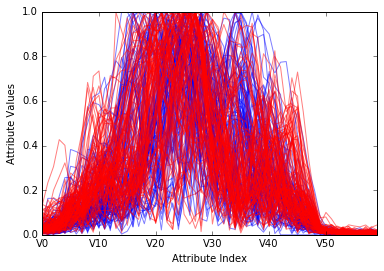no extremely clear separation is evident in the line plot, but there are some areas where the blues and reds are separated. Along the bottom of the plot, the blues stand out a bit, and in the range of attribute indices from 30 to 40, the blues are somewhat higher than the reds. These kinds of insights can help in interpreting and confirming predictions made by your trained model.

# Visualizing Interrelationships between Attributes and Labels

Another question you might ask of the data is how the various attributes relate to one another. One quick way to get an idea of pair‐wise relationships is to crossplot the attributes with the labels.

# Scatter Plot / Cross-Plots

In :
```#calculate correlations between real-valued attributes
dataRow2 = rocksVMines.iloc[1,0:60]
dataRow3 = rocksVMines.iloc[2,0:60]

plot.scatter(dataRow2, dataRow3)

plot.xlabel("2nd Attribute")
plot.ylabel(("3rd Attribute"))
plot.show()

dataRow21 = rocksVMines.iloc[20,0:60]

plot.scatter(dataRow2, dataRow21)

plot.xlabel("2nd Attribute")
plot.ylabel(("21st Attribute"))
plot.show()
```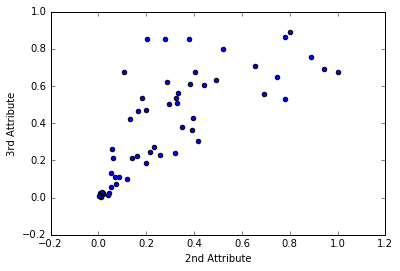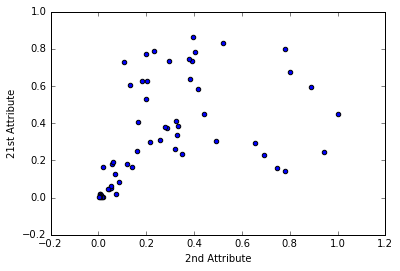If you want to develop your intuition about the relation between numeric correlation and the shape of the scatter plot, just search “correlation”

Basically, if the points in the scatter plot lie along a thin straight line, the two variables are highly correlated; if they form a ball of points, they’re uncorrelated.

# Correlation between Classification Target and Real Attributes

### Plotting a scatter plot between the targets and attribute 35.

The idea of using attribute 35 for the example showing correlation with the target came from the parallel coordinates graph

In :
```from random import uniform
#change the targets to numeric values
target = []
for i in range(208):
#assign 0 or 1 target value based on "M" or "R" labels
if rocksVMines.iat[i,60] == "M":
target.append(1.0)
else:
target.append(0.0)

#plot rows of data as if they were series data
dataRow = rocksVMines.iloc[0:208,35]
plot.scatter(dataRow, target)

plot.xlabel("Attribute Value")
plot.ylabel("Target Value")
plot.show()

#
#To improve the visualization, this version dithers the points a little
# and makes them somewhat transparent
target = []
for i in range(208):
#assign 0 or 1 target value based on "M" or "R" labels
if rocksVMines.iat[i,60] == "M":
target.append(1.0 + uniform(-0.1, 0.1))
else:
target.append(0.0 + uniform(-0.1, 0.1))

#plot rows of data as if they were series data
dataRow = rocksVMines.iloc[0:208,35]
plot.scatter(dataRow, target, alpha=0.5, s=120)

plot.xlabel("Attribute Value")
plot.ylabel("Target Value")
plot.show()
```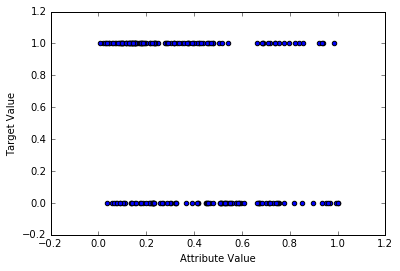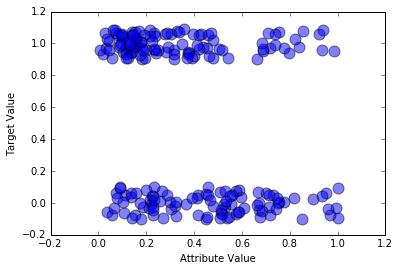Notice the somewhat higher concentration of attribute 35 on the left end of the upper band of points, whereas the points are more uniformly spread from right to left in the lower band. The upper band of points corresponds to mines. The lower band corresponds to rocks. You could build a classifier for this problem by testing whether attribute 35 is greater than or less than 0.5. If it is greater than 0.5 call it a rock and if it is less than 0.5, call it a mine. The examples where attribute 35 is less than 0.5 contain a higher concentration of mines than rock, and the examples where attribute 35 is less than 0.5 contain a lower density, so you’d get better performance than you would with random guessing.

The degree of correlation between two attributes (or an attribute and a target) can be quantified using Pearson’s correlation coefficient.

The attributes that have close index numbers have relatively higher correlations than those that are separated further.

# Pearson’s Correlation Calculation for Attributes 2 versus 3 and 2 versus 21

In :
```from math import sqrt

#calculate correlations between real-valued attributes
dataRow2 = rocksVMines.iloc[1,0:60]
dataRow3 = rocksVMines.iloc[2,0:60]
dataRow21 = rocksVMines.iloc[20,0:60]

mean2 = 0.0; mean3 = 0.0; mean21 = 0.0
numElt = len(dataRow2)
for i in range(numElt):
mean2 += dataRow2[i]/numElt
mean3 += dataRow3[i]/numElt
mean21 += dataRow21[i]/numElt

var2 = 0.0; var3 = 0.0; var21 = 0.0
for i in range(numElt):
var2 += (dataRow2[i] - mean2) * (dataRow2[i] - mean2)/numElt
var3 += (dataRow3[i] - mean3) * (dataRow3[i] - mean3)/numElt
var21 += (dataRow21[i] - mean21) * (dataRow21[i] - mean21)/numElt

corr23 = 0.0; corr221 = 0.0
for i in range(numElt):
corr23 += (dataRow2[i] - mean2) *
(dataRow3[i] - mean3) / (sqrt(var2*var3) * numElt)
corr221 += (dataRow2[i] - mean2) *
(dataRow21[i] - mean21) / (sqrt(var2*var21) * numElt)

sys.stdout.write("Correlation between attribute 2 and 3 n")
print(corr23)
sys.stdout.write(" n")

sys.stdout.write("Correlation between attribute 2 and 21 n")
print(corr221)
sys.stdout.write(" n")
```
```Correlation between attribute 2 and 3
0.770938121191

Correlation between attribute 2 and 21
0.466548080789

```

# Visualizing Attribute and Label Correlations Using a Heat Map

One way to check correlations with a large number of attributes is to calculate the Pearson’s correlation coefficient for pairs of attributes, arrange those correlations into a matrix where the ij‐th entry is the correlation between the ith attribute and the jth attribute, and then plot them in a heat map

In :
```#calculate correlations between real-valued attributes

corMat = DataFrame(rocksVMines.corr())

#visualize correlations using heatmap
plot.pcolor(corMat)
plot.show()
```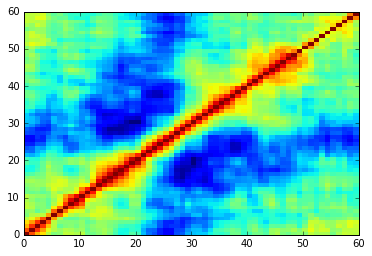The light areas along the diagonal confirm that attributes close to one another in index have relatively high correlations. As mentioned earlier, this is due to the way in which the data are generated. Close indices are sampled at short time intervals from one another and consequently have similar frequencies. Similar frequencies reflect off the targets similarly (and so on).

Perfect correlation (correlation = 1) between attributes means that you may have made a mistake and included the same thing twice. Very high correlation between a set of attributes (pairwise correlations > 0.7) is known as multicollinearity and can lead to unstable estimates. Correlation with the targets is a different matter. Having an attribute that’s correlated with the target generally indicates a predictive relation.

This notebook contains my notes for Predictive Analysis on Binary Classification. It acts as a cookbook. It is a continuation from the previous post on Pearson’s Correlation. This notebook discusses assessing performance of Predictive Models.

One of the most used is the misclassification error—that is, the fraction of examples that the function pred() predicts incorrectly.

In :
```from urllib.request import urlopen
import sys
#read data from uci data repository
target_url = urllib.request.Request("https://archive.ics.uci.edu/ml/machine-learning-databases/undocumented/connectionist-bench/sonar/sonar.all-data")
data = urlopen(target_url)

#arrange data into list for labels and list of lists for attributes
xList = []
labels = []

for line in data:
#split on comma
row = line.strip().decode().split(",")
#assign label 1.0 for "M" and 0.0 for "R"
if(row[-1] == 'M'):
labels.append(1.0)
else:
labels.append(0.0)
#remove label from row
row.pop()
#convert row to floats
floatRow = [float(num) for num in row]
xList.append(floatRow)
```

confusionMatrix() takes the predictions, the corresponding actual values (labels), and a threshold value as input. It compares the predictions to the threshold to determine whether to assign each example to the “predicted positive” or “predicted negative” column in the confusion matrix. It uses the actual value to make the assignment to the appropriate row of the confusion matrix.

In :
```#import random
from sklearn import datasets, linear_model

def confusionMatrix(predicted, actual, threshold):
if len(predicted) != len(actual): return -1
tp = 0.0
fp = 0.0
tn = 0.0
fn = 0.0
for i in range(len(actual)):
if actual[i] > 0.5: #labels that are 1.0  (positive examples)
if predicted[i] > threshold:
tp += 1.0 #correctly predicted positive
else:
fn += 1.0 #incorrectly predicted negative
else:              #labels that are 0.0 (negative examples)
if predicted[i] < threshold:
tn += 1.0 #correctly predicted negative
else:
fp += 1.0 #incorrectly predicted positive
rtn = [tp, fn, fp, tn]
return rtn

#divide attribute matrix and label vector into training(2/3 of data) and test sets (1/3 of data)
indices = range(len(xList))
xListTest = [xList[i] for i in indices if i%3 == 0 ]
xListTrain = [xList[i] for i in indices if i%3 != 0 ]
labelsTest = [labels[i] for i in indices if i%3 == 0]
labelsTrain = [labels[i] for i in indices if i%3 != 0]

#form list of list input into numpy arrays to match input class for scikit-learn linear model
xTrain = np.array(xListTrain); yTrain = np.array(labelsTrain); xTest = np.array(xListTest); yTest = np.array(labelsTest)

#check shapes to see what they look like
print("Shape of xTrain array", xTrain.shape)
print("Shape of yTrain array", yTrain.shape)
print("Shape of xTest array", xTest.shape)
print("Shape of yTest array", yTest.shape)
```
```Shape of xTrain array (138, 60)
Shape of yTrain array (138,)
Shape of xTest array (70, 60)
Shape of yTest array (70,)
```

The data labeled test will not be used in training the classifier, but will be reserved for assessing performance after the classifier is trained.

In :
```#train linear regression model
rocksVMinesModel = linear_model.LinearRegression()
rocksVMinesModel.fit(xTrain,yTrain)

#generate predictions on in-sample error
trainingPredictions = rocksVMinesModel.predict(xTrain)
print("Some values predicted by model", trainingPredictions[0:5], trainingPredictions[-6:-1])
```
```Some values predicted by model [-0.10240253  0.42090698  0.38593034  0.36094537  0.31520494] [ 1.11094176  1.12242751  0.77626699  1.02016858  0.66338081]
```

The classifier is trained by converting the labels M (for mine) and R (for rock) in the original data set into numeric values—1.0 corresponding to mine, and 0.0 corresponding to rock—and then using the ordinary least squares regression to fit a linear model.

Then the trained model is used to generate predictions on the training set and on the test set.

In :
```#generate confusion matrix for predictions on training set (in-sample
confusionMatTrain = confusionMatrix(trainingPredictions, yTrain, 0.50)
#pick threshold value and generate confusion matrix entries
tp = confusionMatTrain; fn = confusionMatTrain; fp = confusionMatTrain; tn = confusionMatTrain

print("tp = " + str(tp) + "tfn = " + str(fn) + "n" + "fp = " + str(fp) + "ttn = " + str(tn) + 'n')

#Misclassification error rate
def errorRate(tp,fn,fp,tn):
#Accuracy
acc = (tp + tn) / (tp + fn + fp + tn)
return 1- acc
```
```tp = 68.0	fn = 6.0
fp = 7.0	tn = 57.0

Miscalssification error rate is : 0.09420289855072461
```

The linear regression model generates numbers that are mostly in the interval between 0.0 and 1.0, but not entirely. The predictions aren’t quite probabilities. They can still be used to generate predicted classifications by comparing to a threshold value.

In :
```#generate predictions on out-of-sample data
testPredictions = rocksVMinesModel.predict(xTest)

#generate confusion matrix from predictions on out-of-sample data
conMatTest = confusionMatrix(testPredictions, yTest, 0.25)
#pick threshold value and generate confusion matrix entries
tp = conMatTest; fn = conMatTest; fp = conMatTest; tn = conMatTest
print("tp = " + str(tp) + "tfn = " + str(fn) + "n" + "fp = " + str(fp) + "ttn = " + str(tn) + 'n')

print("Miscalssification error rate is : " + str(errorRate(tp,fn,fp,tn)))
```
```tp = 34.0	fn = 3.0
fp = 14.0	tn = 19.0

Miscalssification error rate is : 0.24285714285714288
```

confusion matrices for the in-sample data and the out-ofsample data and prints them both out. The misclassification error rate on the in-sample data is about 8 percent, and about 26 percent on the out-ofsample data.

The misclassification error changes when the thresholds are changed.

The best value for the threshold may be the one that minimizes the misclassification error. Sometimes, however, there’s more cost associated with one type of error than with another.

To characterize the overall performance of the classifier instead of using the misclassification error rate for a particular decision threshold. A common technique for doing that is called the receiver operating characteristic or ROC curve

The ROC curve plots the true positive rate (abbreviated TPR) versus the false positive rate (FPR).

TPR is the proportion of positive examples that are correctly classified as positive. FPR is the number of FPs relative to the total number of actual negatives.

In :
```from sklearn.metrics import roc_curve, auc
#generate ROC curve for in-sample

fpr, tpr, thresholds = roc_curve(yTrain,trainingPredictions)
roc_auc = auc(fpr, tpr)
print( 'AUC for in-sample ROC curve: %f' % roc_auc)
```
```AUC for in-sample ROC curve: 0.979519
```
In :
```import pylab as pl
# Plot ROC curve
pl.clf()
pl.plot(fpr, tpr, label='ROC curve (area = %0.2f)' % roc_auc)
pl.plot([0, 1], [0, 1], 'k--')
pl.xlim([0.0, 1.0])
pl.ylim([0.0, 1.0])
pl.xlabel('False Positive Rate')
pl.ylabel('True Positive Rate')
pl.title('In sample ROC rocks versus mines')
pl.legend(loc="lower right")
pl.show()

#generate ROC curve for out-of-sample
fpr, tpr, thresholds = roc_curve(yTest,testPredictions)
roc_auc = auc(fpr, tpr)
print( 'AUC for out-of-sample ROC curve: %f' % roc_auc)

# Plot ROC curve
pl.clf()
pl.plot(fpr, tpr, label='ROC curve (area = %0.2f)' % roc_auc)
pl.plot([0, 1], [0, 1], 'k--')
pl.xlim([0.0, 1.0])
pl.ylim([0.0, 1.0])
pl.xlabel('False Positive Rate')
pl.ylabel('True Positive Rate')
pl.title('Out-of-sample ROC rocks versus mines')
pl.legend(loc="lower right")
pl.show()
```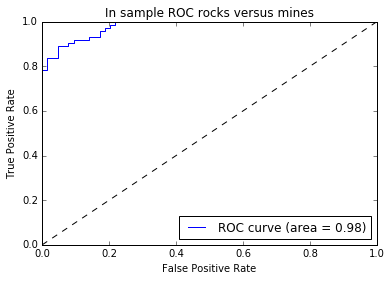```AUC for out-of-sample ROC curve: 0.848485
```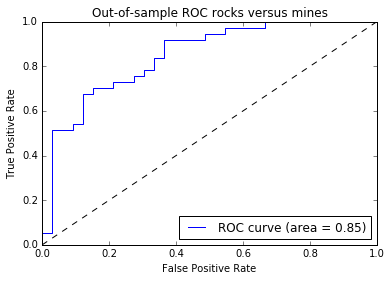For a perfect classifier, the ROC curve steps straight up from (0, 0) to (0, 1) and then goes straight across to (1, 1).

Not surprisingly, in-sample data comes closer to perfection than out-of-sample data.

The closer that a classifier can come to hitting the upper-left corner, the better it is.

If the ROC curve drops significantly below the diagonal line, it usually means that the data scientist has gotten a sign switched somewhere and should examine his code carefully.

A perfect classifier has an AUC of 1.0, and random guessing has an AUC of 0.5.

#### Use of ridge regression with a complexity tuning parameter and AUC as the performance measure for the classifier.

In :
```#form list of list input into numpy arrays to match input class for scikit-learn linear model
xTrain = numpy.array(xListTrain); yTrain = numpy.array(labelsTrain); xTest = numpy.array(xListTest); yTest = numpy.array(labelsTest)

alphaList = [0.1**i for i in [-3, -2, -1, 0,1, 2, 3, 4, 5]]

aucList = []
for alph in alphaList:
rocksVMinesRidgeModel = linear_model.Ridge(alpha=alph)
rocksVMinesRidgeModel.fit(xTrain, yTrain)
fpr, tpr, thresholds = roc_curve(yTest,rocksVMinesRidgeModel.predict(xTest))
roc_auc = auc(fpr, tpr)
aucList.append(roc_auc)

print("AUC             alpha")
for i in range(len(aucList)):
print(aucList[i], alphaList[i])
```
```AUC             alpha
0.841113841114 999.9999999999999
0.864045864046 99.99999999999999
0.907452907453 10.0
0.9180999181 1.0
0.882882882883 0.1
0.861588861589 0.010000000000000002
0.851760851761 0.0010000000000000002
0.850941850942 0.00010000000000000002
0.849303849304 1.0000000000000003e-05
```
In :
```#plot auc values versus alpha values
x = [-3, -2, -1, 0,1, 2, 3, 4, 5]
plot.plot(x, aucList)
plot.xlabel('-log(alpha)')
plot.ylabel('AUC')
plot.show()
```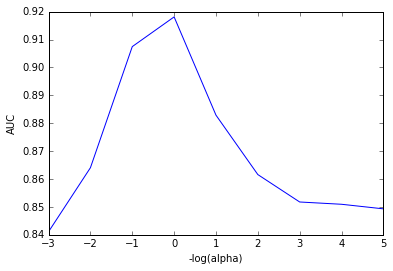In :
```#visualize the performance of the best classifier
indexBest = aucList.index(max(aucList))
alph = alphaList[indexBest]
rocksVMinesRidgeModel = linear_model.Ridge(alpha=alph)
rocksVMinesRidgeModel.fit(xTrain, yTrain)

#scatter plot of actual vs predicted
plot.scatter(rocksVMinesRidgeModel.predict(xTest), yTest, s=100, alpha=0.25)
plot.xlabel("Predicted Value")
plot.ylabel("Actual Value")
plot.show()
```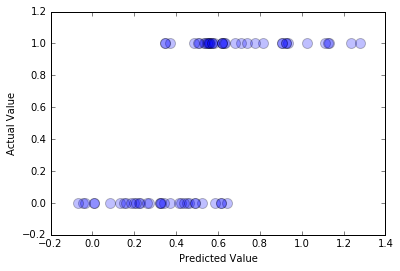# Solving Classification Problems with Penalized Regression

For binary classification problems, you’ll often get good results by coding the binary values as real numbers. This simple procedure codes one of the two binary values as a 1 and the other as a 0 (or +1 and –1). With that simple arrangement, the list of labels becomes a list of real numbers

# Assigning Numeric Values to Binary Labels

In :
```from urllib.request import urlopen
import urllib
#read data from uci data repository
target_url = urllib.request.Request("https://archive.ics.uci.edu/ml/machine-learning-databases/undocumented/connectionist-bench/sonar/sonar.all-data")
data = urlopen(target_url)
#arrange data into list for labels and list of lists for attributes
xList = []
for line in data:
#split on comma
row = line.strip().decode().split(",")
xList.append(row)

#separate labels from attributes, convert from attributes from string to numeric and convert "M" to 1 and "R" to 0

xNum = []
labels = []

for row in xList:
lastCol = row.pop()
if lastCol == "M":
labels.append(1.0)
else:
labels.append(0.0)
attrRow = [float(elt) for elt in row]
xNum.append(attrRow)

#number of rows and columns in x matrix
nrow = len(xNum)
ncol = len(xNum)
```

# Normalization

In :
```#calculate means and variances
xMeans = []
xSD = []
for i in range(ncol):
col = [xNum[j][i] for j in range(nrow)]
mean = sum(col)/nrow
xMeans.append(mean)
colDiff = [(xNum[j][i] - mean) for j in range(nrow)]
sumSq = sum([colDiff[i] * colDiff[i] for i in range(nrow)])
stdDev = sqrt(sumSq/nrow)
xSD.append(stdDev)

#use calculate mean and standard deviation to normalize xNum
xNormalized = []
for i in range(nrow):
rowNormalized = [(xNum[i][j] - xMeans[j])/xSD[j] for j in range(ncol)]
xNormalized.append(rowNormalized)

#Normalize labels
meanLabel = sum(labels)/nrow
sdLabel = sqrt(sum([(labels[i] - meanLabel) * (labels[i] - meanLabel) for i in range(nrow)])/nrow)

labelNormalized = [(labels[i] - meanLabel)/sdLabel for i in range(nrow)]
```
In :
```#initialize a vector of coefficients beta
beta = [0.0] * ncol

#initialize matrix of betas at each step
betaMat = []
betaMat.append(list(beta))

#number of steps to take
nSteps = 350
stepSize = 0.004
nzList = []

for i in range(nSteps):
#calculate residuals
residuals = [0.0] * nrow
for j in range(nrow):
labelsHat = sum([xNormalized[j][k] * beta[k] for k in range(ncol)])
residuals[j] = labelNormalized[j] - labelsHat

#calculate correlation between attribute columns from normalized wine and residual
corr = [0.0] * ncol

for j in range(ncol):
corr[j] = sum([xNormalized[k][j] * residuals[k] for k in range(nrow)]) / nrow

iStar = 0
corrStar = corr

for j in range(1, (ncol)):
if abs(corrStar) < abs(corr[j]):
iStar = j; corrStar = corr[j]

beta[iStar] += stepSize * corrStar / abs(corrStar)
betaMat.append(list(beta))

nzBeta = [index for index in range(ncol) if beta[index] != 0.0]
for q in nzBeta:
if (q in nzList) == False:
nzList.append(q)

#make up names for columns of xNum
names = ['V' + str(i) for i in range(ncol)]
nameList = [names[nzList[i]] for i in range(len(nzList))]

print(nameList)
for i in range(ncol):
#plot range of beta values for each attribute
coefCurve = [betaMat[k][i] for k in range(nSteps)]
xaxis = range(nSteps)
plot.plot(xaxis, coefCurve)

plot.xlabel("Steps Taken")
plot.ylabel(("Coefficient Values"))
plot.show()
```
```['V10', 'V48', 'V44', 'V11', 'V35', 'V51', 'V20', 'V3', 'V21', 'V15', 'V43', 'V0', 'V22', 'V45', 'V53', 'V27', 'V30', 'V50', 'V58', 'V46', 'V56', 'V28', 'V39']
```

# Using ElasticNet Regression

### Cross Validation

In :
```from sklearn.linear_model import enet_path
#alpha = 1.0
#number of cross validation folds
nxval = 10

for ixval in range(nxval):
#Define test and training index sets
idxTest = [a for a in range(nrow) if a%nxval == ixval%nxval]
idxTrain = [a for a in range(nrow) if a%nxval != ixval%nxval]

#Define test and training attribute and label sets
xTrain = numpy.array([xNormalized[r] for r in idxTrain])
xTest = numpy.array([xNormalized[r] for r in idxTest])
labelTrain = numpy.array([labelNormalized[r] for r in idxTrain])
labelTest = numpy.array([labelNormalized[r] for r in idxTest])
alphas, coefs, _ = enet_path(xTrain, labelTrain,l1_ratio=0.8, fit_intercept=False, return_models=False)

#apply coefs to test data to produce predictions and accumulate
if ixval == 0:
pred = numpy.dot(xTest, coefs)
yOut = labelTest
else:
#accumulate predictions
yTemp = numpy.array(yOut)
yOut = numpy.concatenate((yTemp, labelTest), axis=0)

#accumulate predictions
predTemp = numpy.array(pred)
pred = numpy.concatenate((predTemp, numpy.dot(xTest, coefs)), axis = 0)
```
In :
```#calculate miss classification error
misClassRate = []
_,nPred = pred.shape
for iPred in range(1, nPred):
predList = list(pred[:, iPred])
errCnt = 0.0
for irow in range(nrow):
if (predList[irow] < 0.0) and (yOut[irow] >= 0.0):
errCnt += 1.0
elif (predList[irow] >= 0.0) and (yOut[irow] < 0.0):
errCnt += 1.0
misClassRate.append(errCnt/nrow)

#find minimum point for plot and for print
minError = min(misClassRate)
idxMin = misClassRate.index(minError)
plotAlphas = list(alphas[1:len(alphas)])

plot.figure()
plot.plot(plotAlphas, misClassRate, label='Misclassification Error Across Folds', linewidth=2)
plot.axvline(plotAlphas[idxMin], linestyle='--',
label='CV Estimate of Best alpha')
plot.legend()
plot.semilogx()
ax = plot.gca()
ax.invert_xaxis()
plot.xlabel('alpha')
plot.ylabel('Misclassification Error')
plot.axis('tight')
plot.show()
```
In :
```#calculate AUC.
idxPos = [i for i in range(nrow) if yOut[i] > 0.0]
yOutBin =  * nrow
for i in idxPos: yOutBin[i] = 1

auc = []
for iPred in range(1, nPred):
predList = list(pred[:, iPred])
aucCalc = roc_auc_score(yOutBin, predList)
auc.append(aucCalc)

maxAUC = max(auc)
idxMax = auc.index(maxAUC)

plot.figure()
plot.plot(plotAlphas, auc, label='AUC Across Folds', linewidth=2)
plot.axvline(plotAlphas[idxMax], linestyle='--',
label='CV Estimate of Best alpha')
plot.legend()
plot.semilogx()
ax = plot.gca()
ax.invert_xaxis()
plot.xlabel('alpha')
plot.ylabel('Area Under the ROC Curve')
plot.axis('tight')
plot.show()
```
In :
```#plot best version of ROC curve
fpr, tpr, thresh = roc_curve(yOutBin, list(pred[:, idxMax]))
ctClass = [i*0.01 for i in range(101)]

plot.plot(fpr, tpr, linewidth=2)
plot.plot(ctClass, ctClass, linestyle=':')
plot.xlabel('False Positive Rate')
plot.ylabel('True Positive Rate')
plot.show()
```
In :
```print('Best Value of Misclassification Error = ', misClassRate[idxMin])
print('Best alpha for Misclassification Error = ', plotAlphas[idxMin])
print('')
print('Best Value for AUC = ', auc[idxMax])
print('Best alpha for AUC   =  ', plotAlphas[idxMax])

print('')
```
```Best Value of Misclassification Error =  0.22115384615384615
Best alpha for Misclassification Error =  0.0176862447202

Best Value for AUC =  0.868672796508
Best alpha for AUC   =   0.0203348835893

```
In :
```print('Confusion Matrices for Different Threshold Values')

#pick some points along the curve to print.  There are 208 points.  The extremes aren't useful
#Sample at 52, 32 and 6.  Use the calculated values of tpr and fpr along with definitions and
#threshold values.
#Some nomenclature (e.g. see wikkipedia "receiver operating curve")

#P = Positive cases
P = len(idxPos)
#N = Negative cases
N = nrow - P
#TP = True positives = tpr * P
TP = tpr * P
#FN = False negatives = P - TP
FN = P - TP
#FP = False positives = fpr * N
FP = fpr * N
#TN = True negatives = N - FP
TN = N - FP

print('Threshold Value =   ', thresh)
print('TP = ', TP, 'FP = ', FP)
print('FN = ', FN, 'TN = ', TN)

TP = tpr * P; FN = P - TP; FP = fpr * N; TN = N - FP

print('Threshold Value =   ', thresh)
print('TP = ', TP, 'FP = ', FP)
print('FN = ', FN, 'TN = ', TN)

TP = tpr * P; FN = P - TP; FP = fpr * N; TN = N - FP

print('Threshold Value =   ', thresh)
print('TP = ', TP, 'FP = ', FP)
print('FN = ', FN, 'TN = ', TN)
```
```Confusion Matrices for Different Threshold Values
Threshold Value =    -0.486641888022
TP =  108.0 FP =  52.0
FN =  3.0 TN =  45.0
Threshold Value =    -0.158579744682
TP =  92.0 FP =  26.0
FN =  19.0 TN =  71.0
Threshold Value =    0.785342293156
TP =  25.0 FP =  3.0
FN =  86.0 TN =  94.0
```

# For Deployment

Retrain the model on the full data set and pull out the coefficients corresponding to the best alpha—the one determined to minimize out-of-sample error, which is estimated in this case study by cross-validation.

## Coefficient Trajectories for ElasticNet

the l1_ratio variable was set to 0.8, which typically results in more coefficients than Lasso regression, which would correspond to l1_ratio at 1.0.
In :
```#Convert normalized labels to numpy array
Y = numpy.array(labelNormalized)

#Convert normalized attributes to numpy array
X = numpy.array(xNormalized)

alphas, coefs, _ = enet_path(X, Y,l1_ratio=0.4, fit_intercept=False, return_models=False)

plot.plot(alphas,coefs.T)

plot.xlabel('alpha')
plot.ylabel('Coefficients')
plot.axis('tight')
plot.semilogx()
ax = plot.gca()
ax.invert_xaxis()
plot.show()
```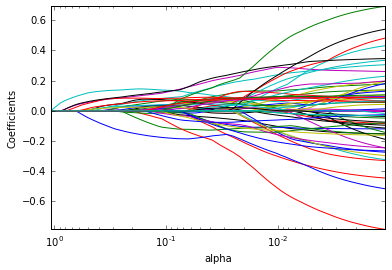In :
```nattr, nalpha = coefs.shape

#find coefficient ordering
nzList = []
for iAlpha in range(1,nalpha):
coefList = list(coefs[: ,iAlpha])
nzCoef = [index for index in range(nattr) if coefList[index] != 0.0]
for q in nzCoef:
if not(q in nzList):
nzList.append(q)

#make up names for columns of X
names = ['V' + str(i) for i in range(ncol)]
nameList = [names[nzList[i]] for i in range(len(nzList))]
print("Attributes Ordered by How Early They Enter the Model")
print(nameList)
print('')
```
```Attributes Ordered by How Early They Enter the Model
['V10', 'V11', 'V48', 'V44', 'V35', 'V47', 'V51', 'V45', 'V20', 'V3', 'V46', 'V50', 'V21', 'V43', 'V0', 'V36', 'V15', 'V27', 'V22', 'V30', 'V53', 'V8', 'V19', 'V56', 'V58', 'V28', 'V39', 'V57', 'V6', 'V14', 'V29', 'V54', 'V7', 'V2', 'V16', 'V49', 'V23', 'V4', 'V37', 'V55', 'V38', 'V13', 'V26', 'V1', 'V31', 'V34', 'V24', 'V33', 'V17', 'V40', 'V41', 'V52', 'V5', 'V59', 'V12', 'V9', 'V18', 'V42', 'V25']

```

One is the order in which variables come into the solution as alpha is decremented downward. The other ordering is according to the magnitude of the coefficients at the optimum solution.

Apparently, V10 is important when the coefficient penalty is so large that the algorithm only permits a single attribute, but when the coefficient penalty has shrunk to the point that a multitude of attributes are included, the attribute V10 levels off and drops in importance somewhat as other attributes are added to the mix.

The value for alpha at which coefficients are sought is hard-coded and comes directly from the results generated previously.

Best alpha for AUC = 0.0203348835893

In :
```#find coefficients corresponding to best alpha value. alpha value corresponding to
#normalized X and normalized Y is 0.020334883589342503

alphaStar = 0.020334883589342503
indexLTalphaStar = [index for index in range(100) if alphas[index] > alphaStar]
indexStar = max(indexLTalphaStar)

#here's the set of coefficients to deploy
coefStar = list(coefs[:,indexStar])
print("Best Coefficient Values ")
print(coefStar)
print('')
```
```Best Coefficient Values
[0.082258256813765362, 0.0020619887220049708, -0.11828642590855749, 0.16633956932499508, 0.0042854388193718151, -0.0, -0.043662524745939846, -0.077515104879432029, 0.10000054356324138, 0.0, 0.090617207036278194, 0.21210870399915979, -0.0, -0.01065538614982325, -0.0, -0.13328659558143635, -0.0, 0.0, 0.0, 0.052814854501417319, 0.038531154796720188, 0.0035515348181845035, 0.090854714680383225, 0.030316113904023091, -0.0, 0.0, 0.008619554235748025, 0.0, 0.0, 0.17497679257272619, -0.2215687804617211, 0.012614243827936522, 0.0, -0.0, 0.0, -0.17160601809439535, -0.080450013824214447, 0.078096790041521466, 0.022035287616765691, -0.072184409273692046, 0.0, -0.0, 0.0, 0.057018816876251224, 0.096478265685718739, 0.039917367637239083, 0.049158231541621127, 0.0, 0.22671917920123977, -0.096272735479952229, 0.0, 0.078886784332226123, 0.0, 0.062312821755756205, -0.082785510713294985, 0.014466967172068062, -0.07432652752563236, 0.068096475974257484, 0.070488864435477985, 0.0]

```
In :
```#The coefficients on normalized attributes give another slightly different ordering

absCoef = [abs(a) for a in coefStar]

#sort by magnitude
coefSorted = sorted(absCoef, reverse=True)

idxCoefSize = [absCoef.index(a) for a in coefSorted if not(a == 0.0)]

namesList2 = [names[idxCoefSize[i]] for i in range(len(idxCoefSize))]

print("Attributes Ordered by Coef Size at Optimum alpha")
print(namesList2)
```
```Attributes Ordered by Coef Size at Optimum alpha
['V48', 'V30', 'V11', 'V29', 'V35', 'V3', 'V15', 'V2', 'V8', 'V44', 'V49', 'V22', 'V10', 'V54', 'V0', 'V36', 'V51', 'V37', 'V7', 'V56', 'V39', 'V58', 'V57', 'V53', 'V43', 'V19', 'V46', 'V6', 'V45', 'V20', 'V23', 'V38', 'V55', 'V31', 'V13', 'V26', 'V4', 'V21', 'V1']
```

# Penalized Logistic Regression – Glmnet

The process has to be iterated until the probabilities (and corresponding weights) stop changing. Basically, the IRLS for logistic regression adds another layer of iteration to the algorithm for (not logistic) penalized regression

In :
```from math import sqrt, fabs, exp

def S(z,gamma):
if gamma >= fabs(z):
return 0.0
if z > 0.0:
return z - gamma
else:
return z + gamma

def Pr(b0,b,x):
n = len(x)
sum = b0
for i in range(n):
sum += b[i]*x[i]
if sum < -100: sum = -100
return 1.0/(1.0 + exp(-sum))
```
In :
```#Do Not Normalize labels but do calculate averages
meanLabel = sum(labels)/nrow
sdLabel = sqrt(sum([(labels[i] - meanLabel) * (labels[i] - meanLabel) for i in range(nrow)])/nrow)

#initialize probabilities and weights
sumWxr = [0.0] * ncol
sumWxx = [0.0] * ncol
sumWr = 0.0
sumW = 0.0

#calculate starting points for betas
for iRow in range(nrow):
p = meanLabel
w = p * (1.0 - p)
#residual for logistic
r = (labels[iRow] - p) / w
x = xNormalized[iRow]
sumWxr = [sumWxr[i] + w * x[i] * r for i in range(ncol)]
sumWxx = [sumWxx[i] + w * x[i] * x[i] for i in range(ncol)]
sumWr = sumWr + w * r
sumW = sumW + w

avgWxr = [sumWxr[i]/nrow for i in range(ncol)]
avgWxx = [sumWxx[i]/nrow for i in range(ncol)]

maxWxr = 0.0
for i in range(ncol):
val = abs(avgWxr[i])
if val > maxWxr:
maxWxr = val

alpha = 0.8

#calculate starting value for lambda
lam = maxWxr/alpha

#this value of lambda corresponds to beta = list of 0's
#initialize a vector of coefficients beta
beta = [0.0] * ncol
beta0 = sumWr/sumW

#initialize matrix of betas at each step
betaMat = []
betaMat.append(list(beta))

beta0List = []
beta0List.append(beta0)

#begin iteration
nSteps = 100
lamMult = 0.93 #100 steps gives reduction by factor of 1000 in lambda (recommended by authors)
nzList = []
for iStep in range(nSteps):
#decrease lambda
lam = lam * lamMult

#Use incremental change in betas to control inner iteration

#set middle loop values for betas = to outer values
# values are used for calculating weights and probabilities
#inner values are used for calculating penalized regression updates

#take pass through data to calculate averages over data require for iteration
#initilize accumulators

betaIRLS = list(beta)
beta0IRLS = beta0
distIRLS = 100.0
#Middle loop to calculate new betas with fixed IRLS weights and probabilities
iterIRLS = 0
while distIRLS > 0.01:
iterIRLS += 1
iterInner = 0.0

betaInner = list(betaIRLS)
beta0Inner = beta0IRLS
distInner = 100.0
while distInner > 0.01:
iterInner += 1
if iterInner > 100: break

#cycle through attributes and update one-at-a-time
#record starting value for comparison
betaStart = list(betaInner)
for iCol in range(ncol):

sumWxr = 0.0
sumWxx = 0.0
sumWr = 0.0
sumW = 0.0

for iRow in range(nrow):
x = list(xNormalized[iRow])
y = labels[iRow]
p = Pr(beta0IRLS, betaIRLS, x)
if abs(p) < 1e-5:
p = 0.0
w = 1e-5
elif abs(1.0 - p) < 1e-5:
p = 1.0
w = 1e-5
else:
w = p * (1.0 - p)

z = (y - p) / w + beta0IRLS + sum([x[i] * betaIRLS[i] for i in range(ncol)])
r = z - beta0Inner - sum([x[i] * betaInner[i] for i in range(ncol)])
sumWxr += w * x[iCol] * r
sumWxx += w * x[iCol] * x[iCol]
sumWr += w * r
sumW += w

avgWxr = sumWxr / nrow
avgWxx = sumWxx / nrow

beta0Inner = beta0Inner + sumWr / sumW
uncBeta = avgWxr + avgWxx * betaInner[iCol]
betaInner[iCol] = S(uncBeta, lam * alpha) / (avgWxx + lam * (1.0 - alpha))

sumDiff = sum([abs(betaInner[n] - betaStart[n]) for n in range(ncol)])
sumBeta = sum([abs(betaInner[n]) for n in range(ncol)])
distInner = sumDiff/sumBeta
#print number of steps for inner and middle loop convergence to monitor behavior
#print(iStep, iterIRLS, iterInner)

#if exit inner while loop, then set betaMiddle = betaMiddle and run through middle loop again.

#Check change in betaMiddle to see if IRLS is converged
a = sum([abs(betaIRLS[i] - betaInner[i]) for i in range(ncol)])
b = sum([abs(betaIRLS[i]) for i in range(ncol)])
distIRLS = a / (b + 0.0001)
dBeta = [betaInner[i] - betaIRLS[i] for i in range(ncol)]
temp = [betaIRLS[i] + gradStep * dBeta[i] for i in range(ncol)]
betaIRLS = list(temp)

beta = list(betaIRLS)
beta0 = beta0IRLS
betaMat.append(list(beta))
beta0List.append(beta0)

nzBeta = [index for index in range(ncol) if beta[index] != 0.0]
for q in nzBeta:
if not(q in nzList):
nzList.append(q)

#make up names for columns of xNum
names = ['V' + str(i) for i in range(ncol)]
nameList = [names[nzList[i]] for i in range(len(nzList))]

print("Attributes Ordered by How Early They Enter the Model")
print(nameList)
for i in range(ncol):
#plot range of beta values for each attribute
coefCurve = [betaMat[k][i] for k in range(nSteps)]
xaxis = range(nSteps)
plot.plot(xaxis, coefCurve)

plot.xlabel("Steps Taken")
plot.ylabel("Coefficient Values")
plot.show()
```
```Attributes Ordered by How Early They Enter the Model
['V10', 'V48', 'V44', 'V11', 'V35', 'V51', 'V20', 'V3', 'V21', 'V43', 'V50', 'V15', 'V22', 'V27', 'V0', 'V30', 'V53', 'V58', 'V56', 'V6', 'V28', 'V36', 'V39', 'V47', 'V19', 'V7', 'V49', 'V54', 'V2', 'V8', 'V29', 'V38', 'V23', 'V57', 'V13', 'V32', 'V31', 'V42', 'V59', 'V37', 'V45', 'V52', 'V16', 'V25', 'V46', 'V18', 'V33', 'V1', 'V55', 'V4', 'V17', 'V26', 'V12', 'V5', 'V40', 'V34', 'V24', 'V41', 'V9', 'V14']
```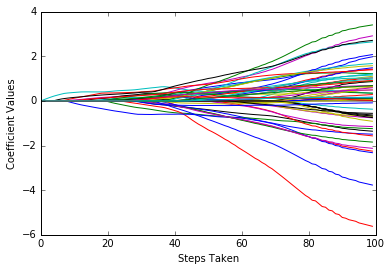## Classifying using Random Forest

In :
```from sklearn.cross_validation import train_test_split
from sklearn import ensemble

#number of rows and columns in x matrix
nrows = len(xNum)
ncols = len(xNum)

#form x and y into numpy arrays and make up column names
X = np.array(xNum)
y = np.array(labels)
rocksVMinesNames = np.array(['V' + str(i) for i in range(ncols)])

#break into training and test sets.
xTrain, xTest, yTrain, yTest = train_test_split(X, y, test_size=0.30, random_state=531)

auc = []

nTreeList = range(50, 2000, 50)
for iTrees in nTreeList:
depth = None
maxFeat  = 8 #try tweaking
rocksVMinesRFModel = ensemble.RandomForestClassifier(n_estimators=iTrees, max_depth=depth, max_features=maxFeat,
oob_score=False, random_state=531)

rocksVMinesRFModel.fit(xTrain,yTrain)

#Accumulate auc on test set
prediction = rocksVMinesRFModel.predict_proba(xTest)
aucCalc = roc_auc_score(yTest, prediction[:,1:2])
auc.append(aucCalc)

print("AUC" )
print(auc[-1])
```
```AUC
0.945740365112
```
In :
```#plot training and test errors vs number of trees in ensemble
plot.plot(nTreeList, auc)
plot.xlabel('Number of Trees in Ensemble')
plot.ylabel('Area Under ROC Curve - AUC')
#plot.ylim([0.0, 1.1*max(mseOob)])
plot.show()
```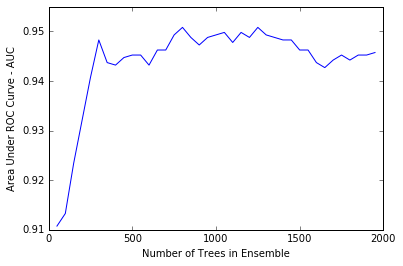For AUC, 1.0 is perfect, and 0.5 is perfectly bad. So, with AUC, larger is better, and instead of looking for a valley in the plot, you’re looking for a peak.

Figure shows a peak toward the left side of the plot.

However, because Random Forest only reduces variance and does not overfit, the peak can be attributed to random fluctuation.

The best choice of model is the one including all the trees whose performance is the rightmost point on the curve.

In :
```# Plot feature importance
featureImportance = rocksVMinesRFModel.feature_importances_
# normalize by max importance
featureImportance = featureImportance / featureImportance.max()

#plot importance of top 30
idxSorted = numpy.argsort(featureImportance)[30:60]
idxTemp = numpy.argsort(featureImportance)[::-1]
print(idxTemp)
barPos = numpy.arange(idxSorted.shape) + .5
plot.barh(barPos, featureImportance[idxSorted], align='center')
plot.yticks(barPos, rocksVMinesNames[idxSorted])
plot.xlabel('Variable Importance')
plot.show()
```
```[10 11  8 20  9 51 48 19 42 47  0 12 35 46  3 16 44  4 45 36 27 26 15 43 50
5 22 18 38 41 34 17 21 14 25  1 53  7  6 13 39 23 52 30 54 31 24 33 32  2
28 37 49 29 57 58 40 59 55 56]
```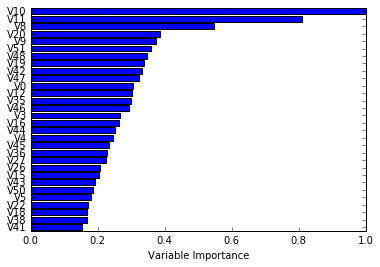The different attributes in the mine detection problem correspond to different frequencies of sonar signal and therefore different wavelengths. If you were given the problem of designing the machine learning for this problem, your next step might be to determine the wavelengths corresponding to these variables and compare those wavelengths to the characteristic dimensions of the rocks and mines in the test and training set.

In :
```#plot best version of ROC curve
fpr, tpr, thresh = roc_curve(yTest, list(prediction[:,1:2]))
ctClass = [i*0.01 for i in range(101)]

plot.plot(fpr, tpr, linewidth=2)
plot.plot(ctClass, ctClass, linestyle=':')
plot.xlabel('False Positive Rate')
plot.ylabel('True Positive Rate')
plot.show()
```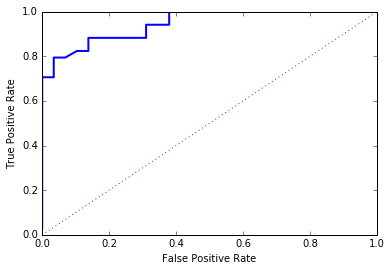In :
```#pick some threshold values and calc confusion matrix for best predictions
#notice that GBM predictions don't fall in range of (0, 1)
#pick threshold values at 25th, 50th and 75th percentiles
idx25 = int(len(thresh) * 0.25)
idx50 = int(len(thresh) * 0.50)
idx75 = int(len(thresh) * 0.75)

#calculate total points, total positives and total negatives
totalPts = len(yTest)
P = sum(yTest)
N = totalPts - P

print('')
print('Confusion Matrices for Different Threshold Values')

#25th
TP = tpr[idx25] * P; FN = P - TP; FP = fpr[idx25] * N; TN = N - FP
print('')
print('Threshold Value =   ', thresh[idx25])
print('TP = ', TP/totalPts, 'FP = ', FP/totalPts)
print('FN = ', FN/totalPts, 'TN = ', TN/totalPts)

#50th
TP = tpr[idx50] * P; FN = P - TP; FP = fpr[idx50] * N; TN = N - FP
print('')
print('Threshold Value =   ', thresh[idx50])
print('TP = ', TP/totalPts, 'FP = ', FP/totalPts)
print('FN = ', FN/totalPts, 'TN = ', TN/totalPts)

#75th
TP = tpr[idx75] * P; FN = P - TP; FP = fpr[idx75] * N; TN = N - FP
print('')
print('Threshold Value =   ', thresh[idx75])
print('TP = ', TP/totalPts, 'FP = ', FP/totalPts)
print('FN = ', FN/totalPts, 'TN = ', TN/totalPts)
```
```Confusion Matrices for Different Threshold Values

Threshold Value =    0.636923076923
TP =  0.428571428571 FP =  0.015873015873
FN =  0.111111111111 TN =  0.444444444444

Threshold Value =    0.625128205128
TP =  0.444444444444 FP =  0.0634920634921
FN =  0.0952380952381 TN =  0.396825396825

Threshold Value =    0.557435897436
TP =  0.507936507937 FP =  0.142857142857
FN =  0.031746031746 TN =  0.31746031746
```

# Detecting Unexploded Mines with Python Gradient Boosting

In :
```#form x and y into numpy arrays and make up column names
X = numpy.array(xNum)
y = numpy.array(labels)
rockVMinesNames = numpy.array(['V' + str(i) for i in range(ncols)])

#break into training and test sets.
xTrain, xTest, yTrain, yTest = train_test_split(X, y, test_size=0.30, random_state=531)

#instantiate model
nEst = 2000
depth = 3
learnRate = 0.007
maxFeatures = 20
learning_rate=learnRate,
max_features=maxFeatures)
#train
rockVMinesGBMModel.fit(xTrain, yTrain)

# compute auc on test set as function of ensemble size
auc = []
aucBest = 0.0
predictions = rockVMinesGBMModel.staged_decision_function(xTest)
for p in predictions:
aucCalc = roc_auc_score(yTest, p)
auc.append(aucCalc)

#capture best predictions
if aucCalc > aucBest:
aucBest = aucCalc
pBest = p

idxBest = auc.index(max(auc))

#print best values
print("Best AUC" )
print(auc[idxBest])
print("Number of Trees for Best AUC")
print(idxBest)
```
```Best AUC
0.958417849899
Number of Trees for Best AUC
1163
```
In :
```#plot training deviance and test auc's vs number of trees in ensemble
plot.figure()
plot.plot(range(1, nEst + 1), rockVMinesGBMModel.train_score_, label='Training Set Deviance', linestyle=":")
plot.plot(range(1, nEst + 1), auc, label='Test Set AUC')
plot.legend(loc='upper right')
plot.xlabel('Number of Trees in Ensemble')
plot.ylabel('Deviance / AUC')
plot.show()
```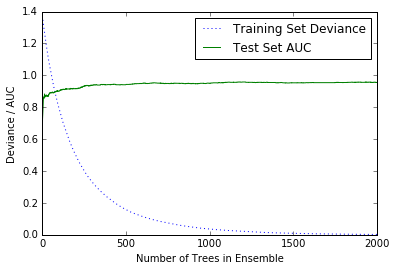Deviance is related to how far the probability estimates are from correct but differs slightly from misclassification error. Deviance is plotted because that quantity is what gradient boosting is training to improve. It’s included in the plot to show the progress of training. The AUC (on oos data) is also plotted to show how the oos performance is changing as the number of trees increases (or equivalently more gradient steps are taken; each step results in training an additional tree).

In :
```# Plot feature importance
featureImportance = rockVMinesGBMModel.feature_importances_

# normalize by max importance
featureImportance = featureImportance / featureImportance.max()

#plot importance of top 30
idxSorted = numpy.argsort(featureImportance)[30:60]

barPos = numpy.arange(idxSorted.shape) + .5
plot.barh(barPos, featureImportance[idxSorted], align='center')
plot.yticks(barPos, rockVMinesNames[idxSorted])
plot.xlabel('Variable Importance')
plot.show()
```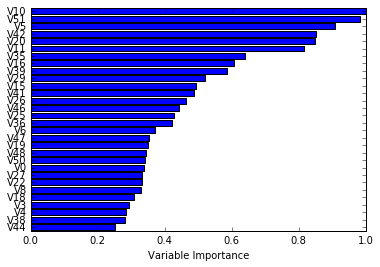The variable importances have a somewhat different order than the ones for Random Forest.

There is some commonality; for example, variables V10, V11, V20 and V51 are near the top of both lists although not in quite the same order.

In :
```#pick some threshold values and calc confusion matrix for best predictions
#notice that GBM predictions don't fall in range of (0, 1)

#plot best version of ROC curve
fpr, tpr, thresh = roc_curve(yTest, list(pBest))
ctClass = [i*0.01 for i in range(101)]

plot.plot(fpr, tpr, linewidth=2)
plot.plot(ctClass, ctClass, linestyle=':')
plot.xlabel('False Positive Rate')
plot.ylabel('True Positive Rate')
plot.show()
```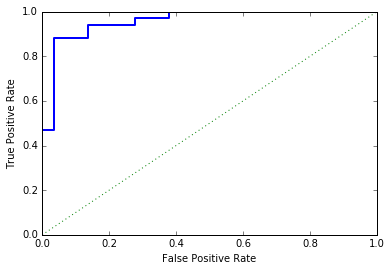Gradient Boosting does get better results using Random Forest base learners, but the difference isn’t large enough to be obvious in the graph.

In :
```#pick some threshold values and calc confusion matrix for best predictions
#notice that GBM predictions don't fall in range of (0, 1)
#pick threshold values at 25th, 50th and 75th percentiles
idx25 = int(len(thresh) * 0.25)
idx50 = int(len(thresh) * 0.50)
idx75 = int(len(thresh) * 0.75)

#calculate total points, total positives and total negatives
totalPts = len(yTest)
P = sum(yTest)
N = totalPts - P

print('')
print('Confusion Matrices for Different Threshold Values')

#25th
TP = tpr[idx25] * P; FN = P - TP; FP = fpr[idx25] * N; TN = N - FP
print('')
print('Threshold Value =   ', thresh[idx25])
print('TP = ', TP/totalPts, 'FP = ', FP/totalPts)
print('FN = ', FN/totalPts, 'TN = ', TN/totalPts)

#50th
TP = tpr[idx50] * P; FN = P - TP; FP = fpr[idx50] * N; TN = N - FP
print('')
print('Threshold Value =   ', thresh[idx50])
print('TP = ', TP/totalPts, 'FP = ', FP/totalPts)
print('FN = ', FN/totalPts, 'TN = ', TN/totalPts)

#75th
TP = tpr[idx75] * P; FN = P - TP; FP = fpr[idx75] * N; TN = N - FP
print('')
print('Threshold Value =   ', thresh[idx75])
print('TP = ', TP/totalPts, 'FP = ', FP/totalPts)
print('FN = ', FN/totalPts, 'TN = ', TN/totalPts)
```
```Confusion Matrices for Different Threshold Values

Threshold Value =    3.73803211613
TP =  0.253968253968 FP =  0.015873015873
FN =  0.285714285714 TN =  0.444444444444

Threshold Value =    0.884206627856
TP =  0.507936507937 FP =  0.0634920634921
FN =  0.031746031746 TN =  0.396825396825

Threshold Value =    0.200107411905
TP =  0.52380952381 FP =  0.174603174603
FN =  0.015873015873 TN =  0.285714285714```

Reference

http://eu.wiley.com/WileyCDA/WileyTitle/productCd-1118961749.html

1. Gabriel